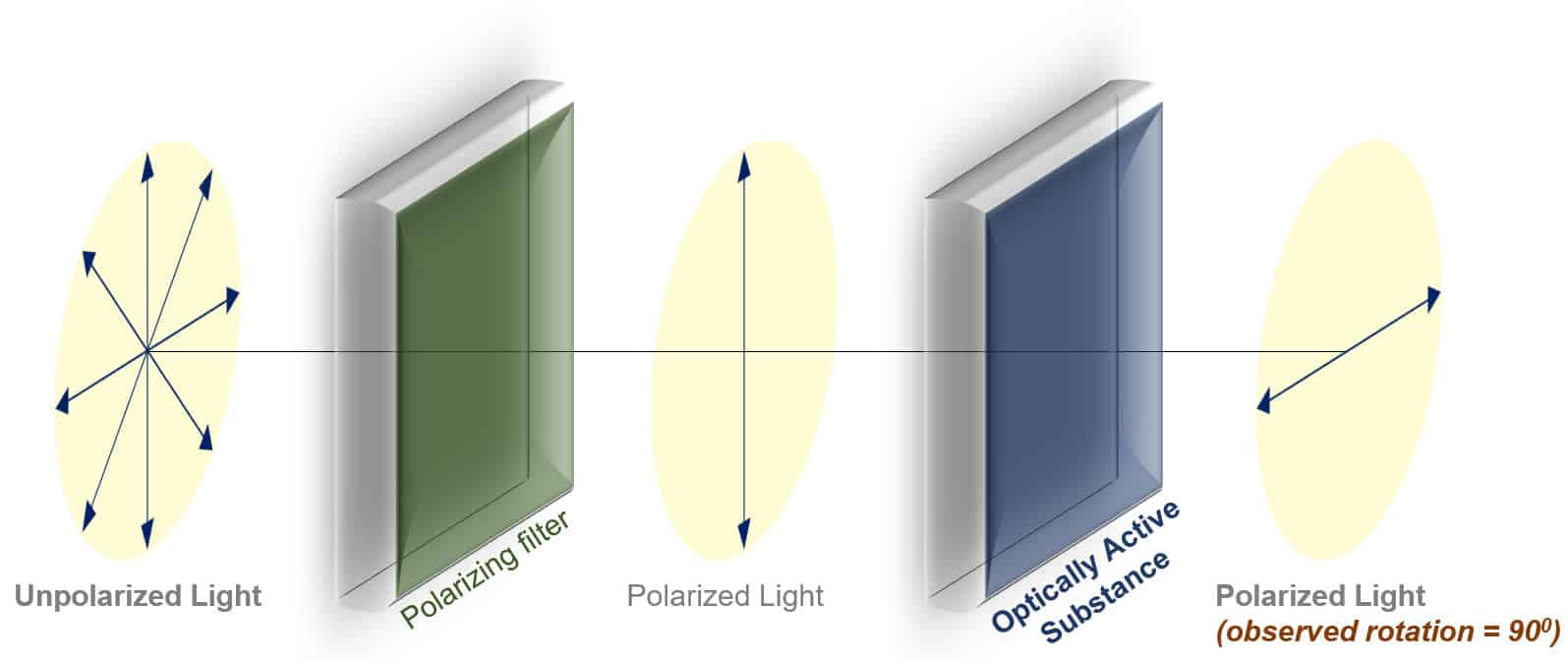# Observed Rotation

## Observed Rotation Definition:

The extent to which plane-polarized light is rotated by a solution of an optically active compound is called the observed rotation.

## Observed Rotation Explained:

The measured/observed rotation of plane-polarized when passing through an optically active compound depends on various factors.

• Concentration – Observed rotation is directly proportional to the concentration of the solution .i.e the number of molecules that the light has to encounter. doubling the concentration doubles the amount of rotation.
• Path length – Observed rotation is also directly proportional to the amount of solution (horizontal distance) that the light has to pass through. Doubling the path length doubles the amount of rotation.

Temperature and the wavelength of the incident light also affect the observed rotation. However, it is not incorporated into the equation below because the relationship is non-linear and unpredictable. Hence, the rotation is always reported along with the temp. and wavelength of the incident light.